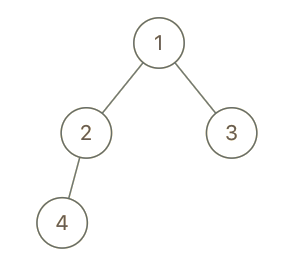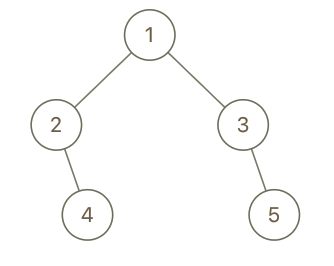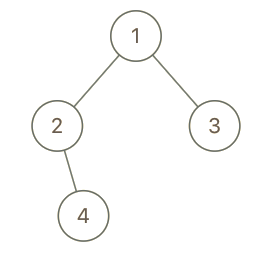# Question

Formatted question description: https://leetcode.ca/all/993.html

In a binary tree, the root node is at depth 0, and children of each depth k node are at depth k+1.

Two nodes of a binary tree are cousins if they have the same depth, but have different parents.

We are given the root of a binary tree with unique values, and the values x and y of two different nodes in the tree.

Return true if and only if the nodes corresponding to the values x and y are cousins.

Example 1:Input: root = [1,2,3,4], x = 4, y = 3
Output: false
Example 2:Input: root = [1,2,3,null,4,null,5], x = 5, y = 4
Output: true
Example 3:Input: root = [1,2,3,null,4], x = 2, y = 3
Output: false
Constraints:

The number of nodes in the tree will be between 2 and 100.
Each node has a unique integer value from 1 to 100.


# Algorithm 1

This question defines a binary tree number of cousin nodes, that is, they do not belong to the same parent node, but have the same depth. Now two node values are given and asked whether the nodes they represent are cousin nodes. Since the cousin nodes must belong to the same level, the level order traversal of the binary tree can be used, just like the previous Binary Tree Level Order Traversal.

Two additional boolean variables isX and isY are needed here to record whether x and y have been traversed. Since it is a layer sequence traversal, a for loop is needed in the while. In the loop, take the first node of the line and see if the node value is equal to x or y. If yes, mark the Boolean variable. Then check whether the left and right child nodes of the current node are x and y respectively, if yes, return false directly. Otherwise, the left and right child nodes are queued, if they exist. After the current layer is traversed, check the values of isX and isY, if both are true, it means that there is a cousin node, and return true. If only one is true, it means that the two are not in the same layer and return false directly. See the code as follows:

# Code 1

C++

class Solution {
public:
bool isCousins(TreeNode* root, int x, int y) {
queue<TreeNode*> q{ {root} };
bool isX = false, isY = false;
while (!q.empty()) {
for (int i = q.size(); i > 0; --i) {
TreeNode *cur = q.front(); q.pop();
if (cur->val == x) isX = true;
if (cur->val == y) isY = true;
if (cur->left && cur->right) {
int left = cur->left->val, right = cur->right->val;
if ((left == x && right == y) || (left == y && right == x)) return false;
}
if (cur->left) q.push(cur->left);
if (cur->right) q.push(cur->right);
}
if (isX && isY) return true;
if (isX || isY) return false;
}
return false;
}
};



# Algorithm 2

Of course, we can also use the recursive method. Since we cannot process the nodes of the same layer at the same time, we need two variables depthX and depthY to record the depth of the nodes x and y, and use a Boolean variable sameParent to record these two Whether the two nodes have the same parent node. In the recursive function, if the current node node is empty, return directly. If the current node value is x, then depthX is assigned to the current depth cur. Similarly, if the current node value is y, then depthY is assigned to the current depth cur. Then check whether the left and right child nodes of the current node are x and y respectively. If yes, mark the sameParent as true, and finally call recursion on the left and right child nodes respectively, see the code as follows:

# Code 2

C++

class Solution {
public:
bool isCousins(TreeNode* root, int x, int y) {
int depthX = 0, depthY = 0;
bool sameParent = false;
helper(root, x, y, 0, depthX, depthY, sameParent);
return !sameParent && depthX == depthY;
}
void helper(TreeNode* node, int x, int y, int cur, int& depthX, int& depthY, bool& sameParent) {
if (!node) return;
if (node->val == x) depthX = cur;
if (node->val == y) depthY = cur;
if (node->left && node->right) {
int left = node->left->val, right = node->right->val;
if ((left == x && right == y) || (left == y && right == x)) sameParent = true;
}
helper(node->left, x, y, cur + 1, depthX, depthY, sameParent);
helper(node->right, x, y, cur + 1, depthX, depthY, sameParent);
}
};



# Algorithm 3

The following method is relatively simple. It is to establish a mapping between each node and the pair composed of its depth and the parent node, and then you can directly use x and y can get its depth and compare with the parent node, see the code as follows:

# Code 3

C++

class Solution {
public:
bool isCousins(TreeNode* root, int x, int y) {
unordered_map<int, pair<int, int>> m;
helper(root, 0, -1, m);
return m[x].first == m[y].first && m[x].second != m[y].second;
}
void helper(TreeNode* node, int depth, int parent, unordered_map<int, pair<int, int>>& m) {
if (!node) return;
m[node->val] = {depth, parent};
helper(node->left, depth + 1, node->val, m);
helper(node->right, depth + 1, node->val, m);
}
};


• /**
* Definition for a binary tree node.
* public class TreeNode {
*     int val;
*     TreeNode left;
*     TreeNode right;
*     TreeNode(int x) { val = x; }
* }
*/
class Solution {
public boolean isCousins(TreeNode root, int x, int y) {
if (root == null || x == y)
return false;
TreeNode xParent = null, yParent = null;
int xDepth = -1, yDepth = -1;
nodeQueue.offer(root);
depthQueue.offer(0);
while (!nodeQueue.isEmpty() && (xDepth < 0 || yDepth < 0)) {
TreeNode node = nodeQueue.poll();
int depth = depthQueue.poll();
TreeNode left = node.left, right = node.right;
if (left != null) {
nodeQueue.offer(left);
depthQueue.offer(depth + 1);
if (left.val == x) {
xParent = node;
xDepth = depth + 1;
}
if (left.val == y) {
yParent = node;
yDepth = depth + 1;
}
}
if (right != null) {
nodeQueue.offer(right);
depthQueue.offer(depth + 1);
if (right.val == x) {
xParent = node;
xDepth = depth + 1;
}
if (right.val == y) {
yParent = node;
yDepth = depth + 1;
}
}
}
return xDepth == yDepth && xParent != yParent;
}
}

############

/**
* Definition for a binary tree node.
* public class TreeNode {
*     int val;
*     TreeNode left;
*     TreeNode right;
*     TreeNode() {}
*     TreeNode(int val) { this.val = val; }
*     TreeNode(int val, TreeNode left, TreeNode right) {
*         this.val = val;
*         this.left = left;
*         this.right = right;
*     }
* }
*/
class Solution {
private int x, y;
private TreeNode p1, p2;
private int d1, d2;

public boolean isCousins(TreeNode root, int x, int y) {
this.x = x;
this.y = y;
dfs(root, null, 0);
return p1 != p2 && d1 == d2;
}

private void dfs(TreeNode root, TreeNode p, int d) {
if (root == null) {
return;
}
if (root.val == x) {
p1 = p;
d1 = d;
}
if (root.val == y) {
p2 = p;
d2 = d;
}
dfs(root.left, root, d + 1);
dfs(root.right, root, d + 1);
}
}

• // OJ: https://leetcode.com/problems/cousins-in-binary-tree/
// Time: O(N)
// Space: O(N)
class Solution {
public:
bool isCousins(TreeNode* root, int x, int y) {
if (root->val == x || root->val == y) return false;
queue<pair<TreeNode*, TreeNode*>> q;
q.emplace((TreeNode*)NULL, root);
while (q.size()) {
int cnt = q.size();
TreeNode *a = NULL, *b = NULL;
while (cnt--) {
auto p = q.front();
q.pop();
if (p.second->val == x) a = p.first;
if (p.second->val == y) b = p.first;
if (p.second->left) q.emplace(p.second, p.second->left);
if (p.second->right) q.emplace(p.second, p.second->right);
}
if (a || b) return a && b && a != b;
}
return false;
}
};

• # Definition for a binary tree node.
# class TreeNode:
#     def __init__(self, val=0, left=None, right=None):
#         self.val = val
#         self.left = left
#         self.right = right
class Solution:
def isCousins(self, root: Optional[TreeNode], x: int, y: int) -> bool:
def dfs(root, fa, d):
if root is None:
return
if root.val == x:
t = (fa, d)
if root.val == y:
t = (fa, d)
dfs(root.left, root, d + 1)
dfs(root.right, root, d + 1)

t = [None, None]
dfs(root, None, 0)
return t != t and t == t

############

# Definition for a binary tree node.
# class TreeNode(object):
#     def __init__(self, x):
#         self.val = x
#         self.left = None
#         self.right = None

class Solution(object):
def isCousins(self, root, x, y):
"""
:type root: TreeNode
:type x: int
:type y: int
:rtype: bool
"""
self.m = collections.defaultdict(tuple)
self.dfs(root, None, 0)
px, dx = self.m[x]
py, dy = self.m[y]
return dx == dy and px != py

def dfs(self, root, parent, depth):
if not root: return
self.m[root.val] = (parent, depth)
self.dfs(root.left, root, depth + 1)
self.dfs(root.right, root, depth + 1)

• /**
* Definition for a binary tree node.
* type TreeNode struct {
*     Val int
*     Left *TreeNode
*     Right *TreeNode
* }
*/
func isCousins(root *TreeNode, x int, y int) bool {
var p1, p2 *TreeNode
var d1, d2 int
var dfs func(*TreeNode, *TreeNode, int)
dfs = func(root *TreeNode, fa *TreeNode, d int) {
if root == nil {
return
}
if root.Val == x {
p1, d1 = fa, d
}
if root.Val == y {
p2, d2 = fa, d
}
dfs(root.Left, root, d+1)
dfs(root.Right, root, d+1)
}
dfs(root, nil, 0)
return p1 != p2 && d1 == d2
}